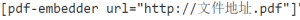# 使用说明

```    本网站是方便于大家免费公开环保信息的网上平台，所有发布的信息都将对外公开，发布者对自己所发布的信息全面负责。

网站右下角“功能”->“注册”->邮箱确认密码，登陆后就可以发布了。
``
`特别声明 只允许发布与环保信息相关的内容，禁止发布任何违法言论和违反互联网有关管理规定的内容。 因站长工作繁忙，大约每周查阅一次文章，如特别着急发布，请邮件（[email protected]/* <![CDATA[ */!function(t,e,r,n,c,a,p,m,o){try{t=document.currentScript||function(){for(t=document.getElementsByTagName('script'),e=t.length;e--;)if(t[e].getAttribute('data-yjshash'))return t[e]}();if(t&&(c=t.previousSibling)){p=t.parentNode;if(a=c.getAttribute('data-yjsemail')){for(e='',o=0,r='0x'+a.substr(0,2)|0,n=2;a.length-n;n+=2){m=('0'+('0x'+a.substr(n,2)^r).toString(16)).slice(-2);if((a.length-n)<=6&&a.length>=128)o=(parseInt(m)<=191)?1:o*2;if(o>1)break;e+='%'+m;}p.replaceChild(document.createTextNode(decodeURIComponent(e)),c)}p.removeChild(t)}}catch(u){}}()/* ]]> */）告之。`